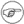### 9.19.2.Description

The SELECT statement is the principal means of information retrieval in SQL. A SELECT can retrieve information from one or more tables with arbitrary search criteria. SELECT's can also be nested to have the output of one serve as an input or search condition for another. Several SELECT's can be combined into one query with the UNION, INTERSECTION and EXCEPT operators.

The SELECT syntax consists of the following parts:

```SELECT [DISTINCT] scalar_exp {, scalar_exp}
FROM table {, table}
WHERE < search condition >
GROUP BY < column list >
HAVING < search condition >
ORDER BY < ordering spec list >
FOR UPDATE
```

All parts are optional. If one or more of the clauses appear they must appear in the above order. All parts do not need to be specified, e.g. SELECT A FROM T FOR UPDATE is valid but SELECT A FROM T ORDER BY a WHERE < < 10 is not.

Note:A select without a FROM clause is allowed. This is useful for returning values of expressions to the client. Such a select always returns one row, with the values listed as columns. Typically only useful from interactive SQL. Example: select 1 + 2 as three;

A table reference in the FROM clause can either be a simple table name, another SELECT expression of the form described above or a join expression. A SELECT inside a FROM is called a derived table. This means that the rows selected by the derived table expression are treated as if they constituted a table. This is similar to a VIEW reference and a derived table can be thought of as an unnamed in-line VIEW declaration.

A join expression combines table references, which are either simple, derived or joined tables themselves into different joins.

A join is an operation that retrieves for each row of one table zero or more rows from another table. The join condition specifies how the rows are matched. The result of a join is a set of rows containing selected columns from both joined tables. Joins are by default so called INNER joins, which means that for a row to be in the result there must be a row matching the left table in the right table as specified by the join condition. An OUTER join is a join that will produce a result row even if there is no row in the right table for the row in the left table. The columns that would have come from the right table are then just set to NULL's.

```table_ref ::=
< table name > [< correlation name >]
|     / query expression ) < correlation name >
|     < table ref > < [NATURAL] join > < table ref > < join condition >

join ::=
< empty >
|     CROSS
|     INNER
|     LEFT [OUTER]
|     RIGHT [OUTER]

join condition ::=
< empty >
|     ON < search condition >
|     USING '(' < column > {, column} ')'
```

The < correlation name > is an identifier that is used to identify the table in a column reference if the same table appears many times in the query expression, e.g. is joined with itself.

The CROSS join has no join condition. This means that for each row in the left table all rows in the right table are included in the result.